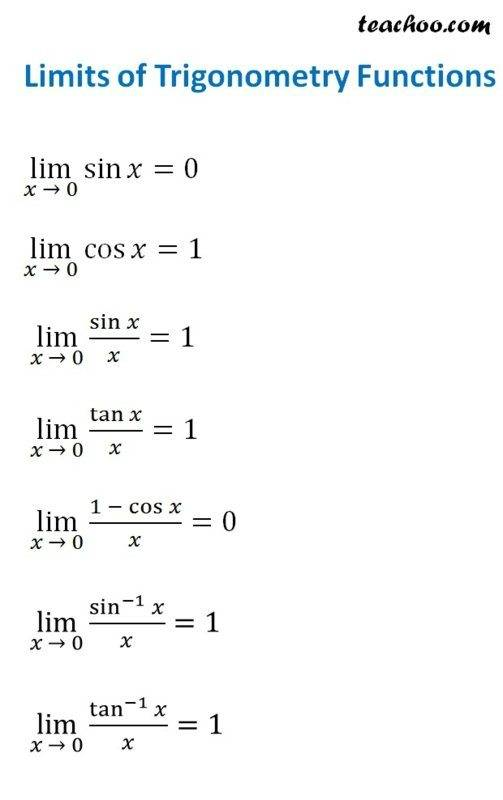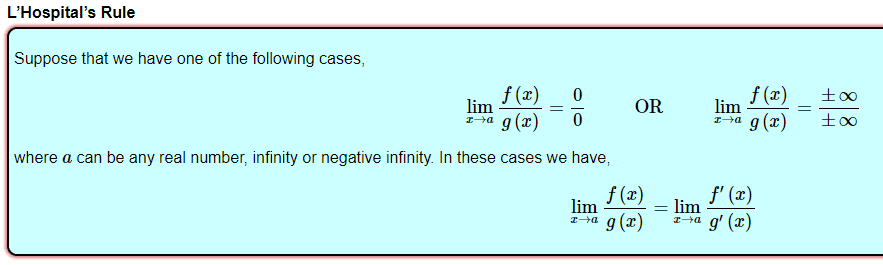# What is my mistake in solving for this limit?

Homework Statement:
Find the limit of the sequence as n approaching infinity.
Relevant Equations:
lim n-->infty n sin (1/n)
I tried to substitution n = infinity so I got (infinity)*sin (1/infinity). I thought 1/infinity is approaching zero. So, sin (1/infinity) is same with sin (0). With these idea, my solution is lim n--> infinity n sin(1/n) = 0.

I tried another method with L'Hopital rule and came with 0/0 form.

So, here is my question:
1. Why my answer isn't valid? Isn't okay that I use sin (1/infinity) = 0?
2. Are there any methods beside the L'Hopital rule and "ln" strategic to find a limit?

Thanks a bunch pals.

member 587159
Heuristically speaking

##\infty.\sin(1/\infty)=\infty.0## is an indeterminate form so you can't proceed like you did.

Rather, try to use l'Hospital's rule.

Since you've tried the 0/0 form, then you probably got this:
$$\lim_{n\to\infty}\frac{\sin\frac 1n}{\frac 1n}$$
How did you apply l'Hôpital's rule? What's ##\frac{d}{dn}(\sin\frac 1n)##?
There're also some limits you might want to know:haruspex
Homework Helper
Gold Member
2020 Award
You might find it helpful to consider x=1/n tending to zero instead.

Heuristically speaking

##\infty.\sin(1/\infty)=\infty.0## is an indeterminate form so you can't proceed like you did.

Rather, try to use l'Hospital's rule.
Yes, I have tried to use L'Hospital's rule and got this:
$$\lim_{n\rightarrow\infty} [{\sin (1/n)}-{\frac {cos (1/n)}{n}}]$$
Is that true?

Then, if I substitute ##\infty## to n I got
$$\sin (1/\infty) - \frac{cos (1/\infty)}{\infty}$$
$$\lim_{n\to\infty}n\sin(1/n)=(0)-\frac{\cos(0)}{\infty}$$

So far I don't see any mistake. But, if I did this, I came up with 0. This is not suitable with answer book, since I trust the answer book, rather then my answer. ..

Please give me insight. Thanks a lot

Since you've tried the 0/0 form, then you probably got this:
$$\lim_{n\to\infty}\frac{\sin\frac 1n}{\frac 1n}$$
How did you apply l'Hôpital's rule? What's ##\frac{d}{dn}(\sin\frac 1n)##?
There're also some limits you might want to know:
View attachment 260823
$$\frac{d}{dn} \sin(1/n)=\sin(1/n)-\frac{cos(1/n)}{n}$$
Is that true?

member 587159
$$\frac{d}{dn} \sin(1/n)=\sin(1/n)-\frac{cos(1/n)}{n}$$
Is that true?

No. What you write is false. You need to apply the chain rule:

$$(f\circ g)'(x) = f'(g(x))g'(x)$$

Here ##g(x) = 1/x##, ##f(x) = \sin(x)##.

No. What you write is false. You need to apply the chain rule:

$$(f\circ g)'(x) = f'(g(x))g'(x)$$

Here ##g(x) = 1/x##, ##f(x) = \sin(x)##.
Ups my mistake. What I write is for ##\frac{d}{dn} (n \sin (1/n))##

So, ##\frac{d}{dn} \sin (1/n)=-\frac{\cos(1/n)}{n}##
Is that any mistake? Thankss

member 587159
Ups my mistake. What I write is for ##\frac{d}{dn} (n \sin (1/n))##

So, ##\frac{d}{dn} \sin (1/n)=-\frac{\cos(1/n)}{n}##
Is that any mistake? Thankss

Still wrong. What is ##\frac{d}{dx}\frac{1}{x}##?

Mark44
Mentor
Ups my mistake. What I write is for ##\frac{d}{dn} (n \sin (1/n))##
For this derivative, you need to use the product rule, which will give you two terms.
agnimusayoti said:
So, ##\frac{d}{dn} \sin (1/n)=-\frac{\cos(1/n)}{n}##
Is that any mistake? Thankss
Yes, that is incorrect. ##\frac{d}{dn} \sin (1/n)= \cos(1/n) \cdot \frac d{dn}(1/n)##. If you carry out the last differentiation what do you get?

The general rule when differentiating powers of ##x## is as follows:
$$\frac{d}{dx}(x^n)=nx^{n-1}$$
How can you write ##1/x## in the form of ##x^n##?

Ah, so I will get -1/n cos (1/n) right? Then, I substitute n = infnty. Is that so? Thankss

Ah, so I will get -1/n cos (1/n) right? Then, I substitute n = infnty. Is that so? Thankss
You've got the minus sign right, but have forgotten to decrease the power.
$$\frac 1x=x^{-1}=x^n$$

You've got the minus sign right, but have forgotten to decrease the power.
$$\frac 1x=x^{-1}=x^n$$
Yeaah, so ##\frac{d}{dn} \sin(1/n)=-1/n^2 \cos(1/n)## I hope it's true

Last edited by a moderator:
•archaic
Yeaah, so ##\frac{d}{dn} \sin(1/n)=-1/n^2 \cos(1/n)## I hope it's true
yeah that's itLast edited by a moderator:
now go back to your limit and plug in the derivatives, something should cancel out.

now go back to your limit and plug in the derivatives, something should cancel out.
If ##f(n)=n\sin(1/n)## then ##f'(n)=\sin(1/n)-\frac{\cos(1/n)}{n}##. Is that true?
Then by L'Hospital's rule; $$\lim_{n\to\infty} n\sin(1/n)=\lim_{n\to\infty} \sin(1/n) - \lim_{n\to\infty} \frac{\cos(1/n)}{n}$$
Can substitute ##n=\infty## to the function so I get:
$$\lim_{n\to\infty} n\sin(1/n)=\sin(1/\infty) - \frac{\cos(1/\infty)}{\infty}$$
$$\lim_{n\to\infty} n\sin(1/n)=\sin(0) - \frac{\cos(0)}{\infty}$$
$$\lim_{n\to\infty} n\sin(1/n)=0 - 0=0$$

Is it true? If that's true, the answer is still different from the key. Hiks. I am not confidence with my answer, though.

haruspex
Homework Helper
Gold Member
2020 Award
Then by L'Hospital's rule;
No, you are not applying it correctly. You must first express the function you want the limit of as the ratio of two functions then differentiate each.

I still think you will find it easier if you replace n with 1/x and consider the limit as x tends to zero.There's also the requirement that ##\lim_{x\to a}\frac{f'(x)}{g'(x)}## exists. If it doesn't, you can either apply the rule again, if possible, or try other methods.

That's the theorem. You need to transform your limit into either of those forms before applying it.

Gee! I think L'Hospital's rule valid for any form. Thanks for
View attachment 260875
There's also the requirement that ##\lim_{x\to a}\frac{f'(x)}{g'(x)}## exists. If it doesn't, you can either apply the rule again, if possible, or try other methods.

That's the theorem. You need to transform your limit into either of those forms before applying it.
So, like haruspex explain, I assume that ##x=1/n## then I will get ##f(n)=n sin(1/n)## equivalent with ##f(x)=\sin(x)/x##

Then, I apply the L'Hospital rule.

So, what's wrong is my concept about L'Hospital rule. Thanks everyone. I will correct my answer.

After I made correction, there's no need to apply L'Hospital because
$$x=1/n$$
so,
$$\lim_{n\to\infty} n \sin(1/n) \equiv \lim_{x\to0} \frac {sin x}{x}$$
By the theorem:
$$\lim_{x\to0} \frac{\sin x}{x}=1$$

Is that true, shifu?

•archaic
Using l'Hôpital's rule:
$$n\sin\frac 1n=\frac{1}{\frac 1n}\sin\frac 1n=\frac{\sin\frac 1n}{\frac 1n}\\ \lim_{n\to\infty}n\sin\frac 1n=\lim_{n\to\infty}\frac{\sin\frac 1n}{\frac 1n}=\lim_{n\to\infty}\frac{-\frac{1}{n^2}\cos\frac 1n}{-\frac{1}{n^2}}=\lim_{n\to\infty}\cos\frac 1n=1$$
Another way is with the substitution you made:
$$\lim_{n\to\infty}n\sin\frac 1n=\lim_{n\to\infty}\frac{\sin\frac 1n}{\frac 1n}=\lim_{x\to0}\frac{\sin x}{x}=\lim_{x\to0}\frac{\cos x}{1}=1$$
Or, also, directly using the theorem as you did.

•agnimusayoti
haruspex
Homework Helper
Gold Member
2020 Award
After I made correction, there's no need to apply L'Hospital because
$$x=1/n$$
so,
$$\lim_{n\to\infty} n \sin(1/n) \equiv \lim_{x\to0} \frac {sin x}{x}$$
By the theorem:
$$\lim_{x\to0} \frac{\sin x}{x}=1$$

Is that true, shifu?
It is not clear whether you are permitted to use that result or, alternatively, you are effectively being asked to prove it.

Last edited:
Using l'Hôpital's rule:
$$n\sin\frac 1n=\frac{1}{\frac 1n}\sin\frac 1n=\frac{\sin\frac 1n}{\frac 1n}\\ \lim_{n\to\infty}n\sin\frac 1n=\lim_{n\to\infty}\frac{\sin\frac 1n}{\frac 1n}=\lim_{n\to\infty}\frac{-\frac{1}{n^2}\cos\frac 1n}{-\frac{1}{n^2}}=\lim_{n\to\infty}\cos\frac 1n=1$$
Another way is with the substitution you made:
$$\lim_{n\to\infty}n\sin\frac 1n=\lim_{n\to\infty}\frac{\sin\frac 1n}{\frac 1n}=\lim_{x\to0}\frac{\sin x}{x}=\lim_{x\to0}\frac{\cos x}{1}=1$$
Or, also, directly using the theorem as you did.
Yes! Thank you. In this case I can use the theorem .

•archaic